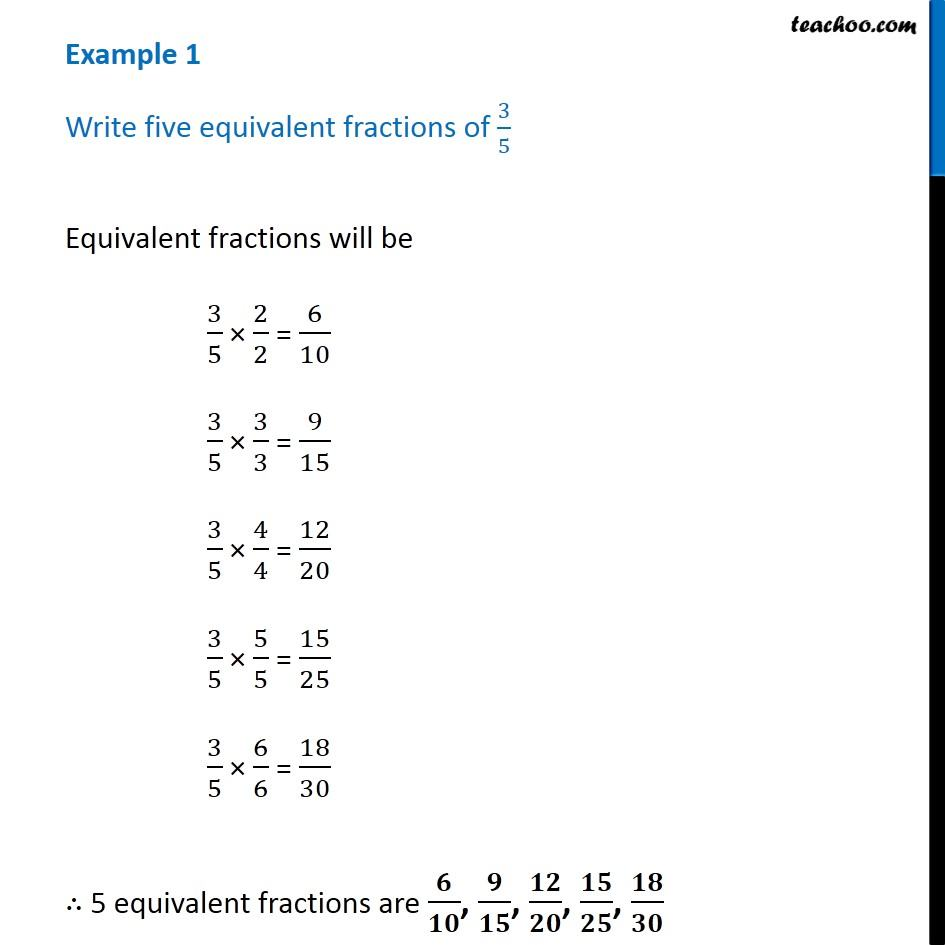Examples

Chapter 2 Class 7 Fractions and Decimals
Serial order wiseLearn in your speed, with individual attention - Teachoo Maths 1-on-1 Class

### Transcript

Question 1 Write five equivalent fractions of 3/5Equivalent fractions will be 3/5 × 2/2 = 6/10 3/5 × 3/3 = 9/15 3/5 × 4/4 = 12/20 3/5 × 5/5 = 15/25 3/5 × 6/6 = 18/30 ∴ 5 equivalent fractions are 𝟔/𝟏𝟎, 𝟗/𝟏𝟓, 𝟏𝟐/𝟐𝟎, 𝟏𝟓/𝟐𝟓, 𝟏𝟖/𝟑𝟎